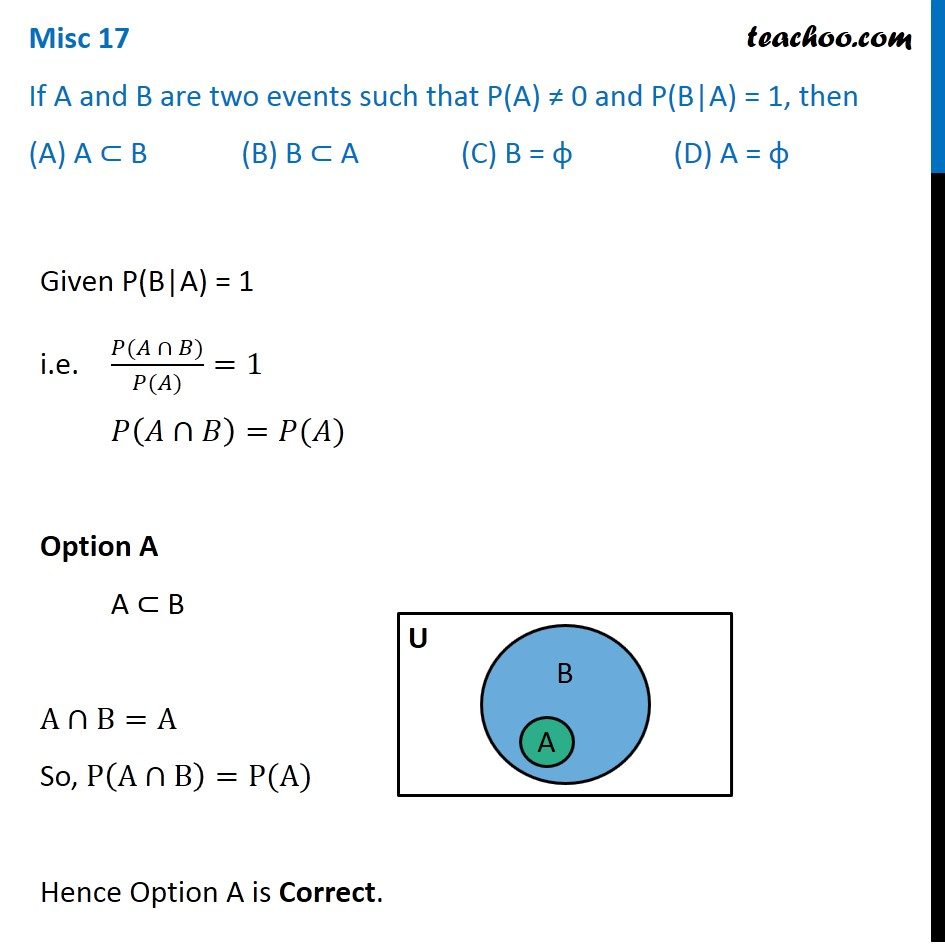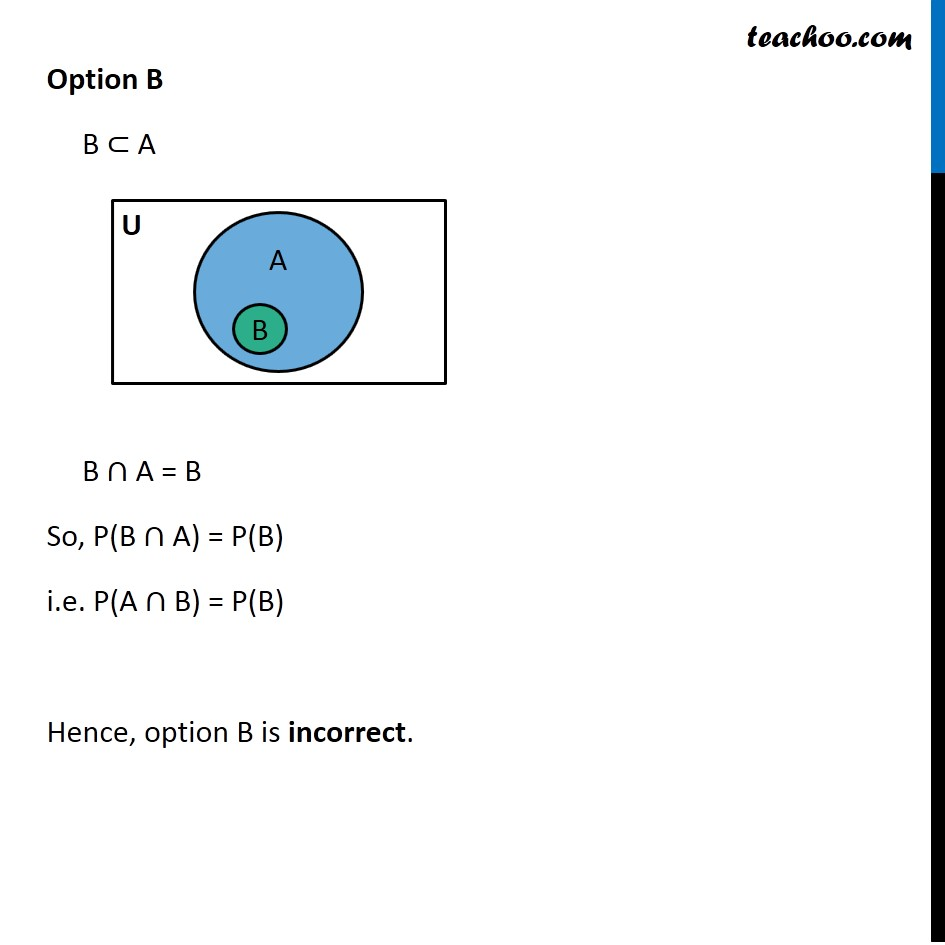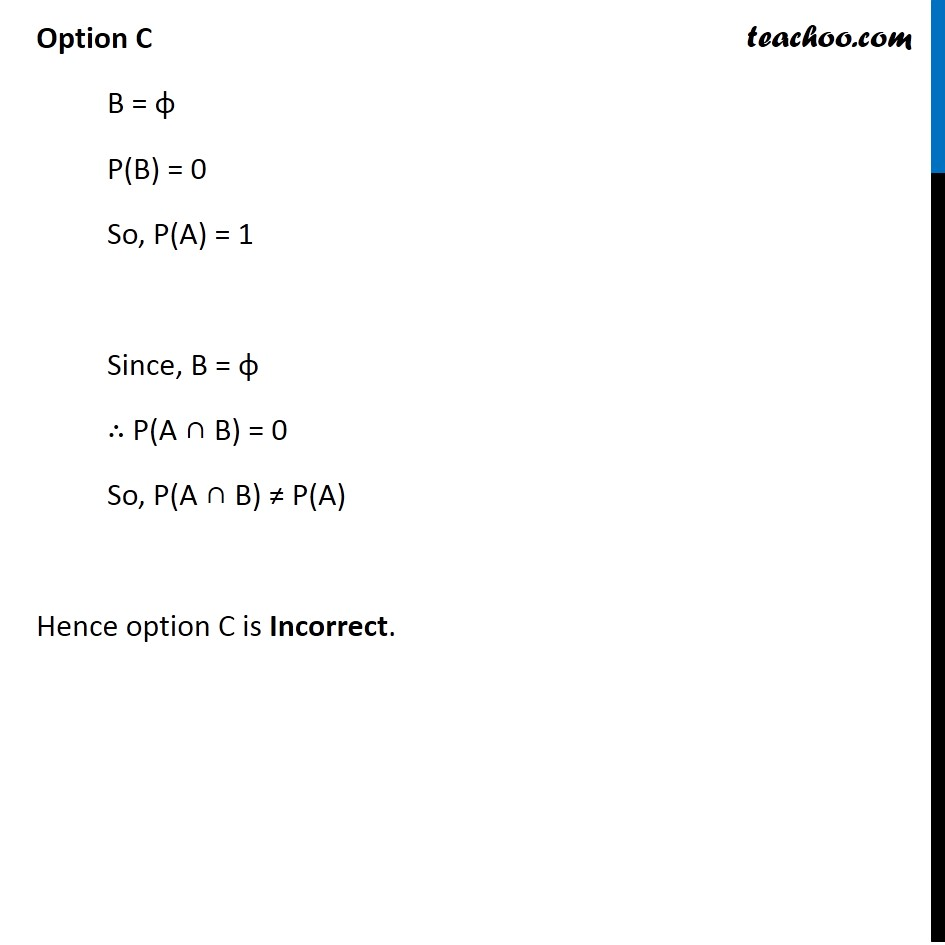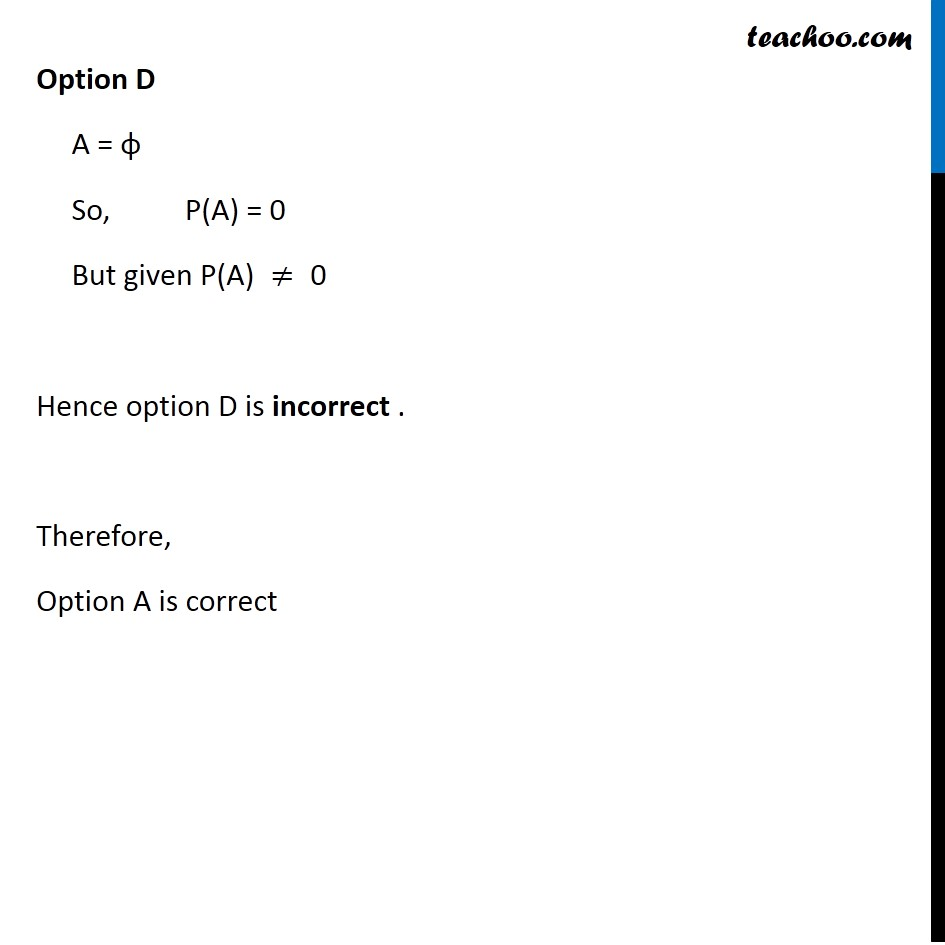Conditional Probability - Values given

Chapter 13 Class 12 Probability
Concept wiseIntroducing your new favourite teacher - Teachoo Black, at only ₹83 per month

### Transcript

Misc 17 If A and B arAe two events such that P(A) ≠ 0 and P(B|A) = 1, then (A) A ⊂ B (B) B ⊂ A (C) B = φ (D) A = φ Given P(B|A) = 1 i.e. (𝑃(𝐴 ∩ 𝐵))/(𝑃(𝐴))=1 𝑃(𝐴∩𝐵)=𝑃(𝐴) Option A A ⊂ B A∩B=A So, P(A∩B)=P(A) Hence Option A is Correct. Option B B ⊂ A B ∩ A = B So, P(B ∩ A) = P(B) i.e. P(A ∩ B) = P(B) Hence, option B is incorrect. Option C B = φ P(B) = 0 So, P(A) = 1 Since, B = φ ∴ P(A ∩ B) = 0 So, P(A ∩ B) ≠ P(A) Hence option C is Incorrect. Option D A = φ So, "P(A) = 0" But given "P(A) "≠" 0" Hence option D is incorrect . Therefore, Option A is correct